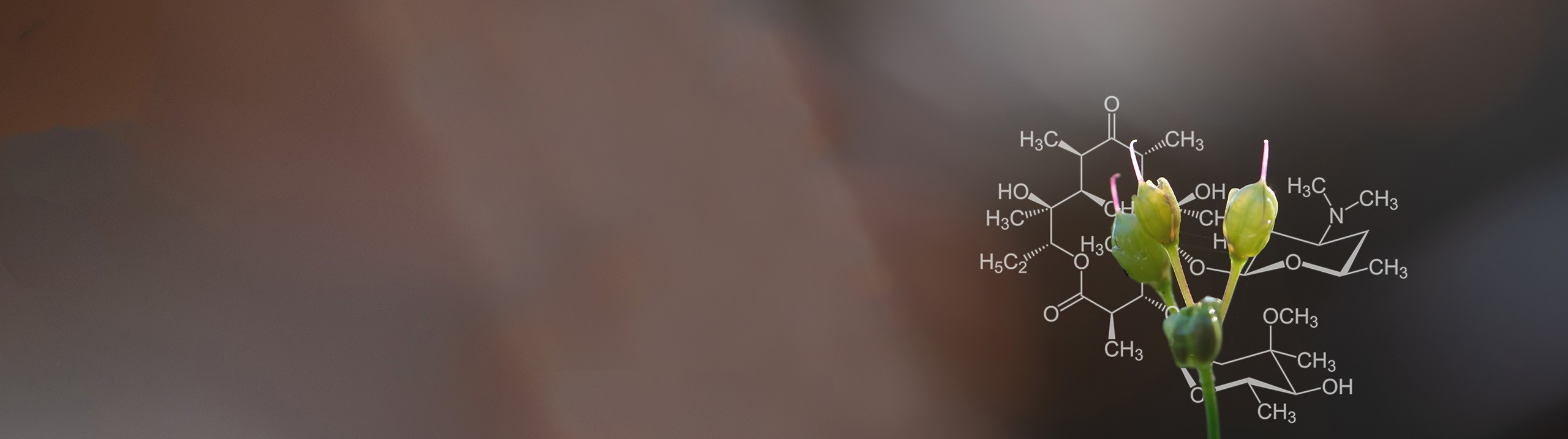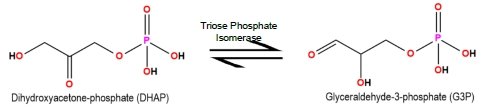Start typing, then use the up and down arrows to select an option from the list.# Biochemistry

Learn the toughest concepts covered in Biochemistry with step-by-step video tutorials and practice problems by world-class tutors

6. Enzymes and Enzyme Kinetics
1

#### concept

Calculating Vmax3m
Play a video:
in this video, we're going to begin our discussion on some of the tools that we have to calculate the theoretical maximal reaction velocity or the V Max. And so it turns out that the V max can actually be calculated in multiple ways. And we're really going to talk about two primary ways to calculate the V Max. And so the first way that the V Max can be calculated is just by algebraic rearrangement. It can be calculated by algebraic rearrangement specifically of the meticulous meant in, or the line Weaver Burke equations and so down below. In our example, we're going to algebraic Lee. Rearrange. The McHale is meant in equation to solve for the V Max, and so notice down below. What we have is the meticulous mental equation right here on the left and on the right. What we have is the line Weaver Burke equation. And so what we need to recall is that the line Weaver Burke equation is literally just the reciprocal of the McHale is meant an equation. And so that means that the relationship between the McHale is meant in, and the line Weaver Burke equations is that they are reciprocal of one another. And so they could be pretty easily, uh, inter converted between each other. And so, essentially, starting over here with the McHale is meant an equation we can solve for the V, max. So we wanna isolate and solve for this variable, and we can start off by essentially just moving this entire denominator here. And so what we could do is just move this denominator up into this side right here. And we could do that by multiplying both sides of our equation here by the denominator k M plus substrate concentration. And what that does is it gets rid of the denominator on the right side. So we're just left with the V Max times the substrate concentration on the right. And then on the left side, all we have is the initial reaction velocity times this denominator. So essentially the initial reaction velocity times, the k m plus the substrate concentration. So now again, we want to continue to isolate and solve for this v Max, just like we're being asked to do in the example. And so what we could do is get rid of this substrate concentration by dividing both sides of the equation by the substrate concentration and so that will get rid of the substrate concentration on the right so that we have V Max all by itself on the right. And of course, we're going to have the same, uh, expression that we had up above. So we're gonna have the initial velocity times, the K M plus the substrate concentration on the top and all divided by the substrate concentration, uh, that we needed to do to get rid of this on the right. And so what, you can see years we've algebraic Lee rearranged. The McHale is meant in equation to solve for the V max. And so this is actually in expression that we can use to solve for the to calculate the V max and some of our practice problems, and so essentially will be able to utilize some of this moving forward in our course. But in our next lesson video, we're going to talk about a second major way that we can use to calculate the V Max. So I'll see you guys in that video
2

#### concept

Calculating Vmax5m
Play a video:
So, in addition to calculating the V Max through algebraic rearrangement of the McHale is meant in and the line Weaver Burke equations. We can also calculate the V Max through rate laws. And so what we need to recall from our previous lesson videos is that the V max is actually directly proportional to both the product formation rate constant, which is K two, and the total enzyme concentration. And so we did cover this in some of our previous lesson videos. But just to refresh our memories, let's recall some information from our previous lesson videos and so recall that biochemist mainly focus on measuring and plotting the initial reaction velocity, or the V not of enzyme catalyzed reactions. And so we know that the initial reaction velocity or the V not is also expressed as the change in the concentration of product over the change in time. And so because it's the product concentration that is relevant to biochemist, they actually focus on measuring the initial reaction velocity of the product formation step in our typical enzyme catalyzed reactions, and so notice down below. What we have is our typical enzyme catalyzed reaction, and we know that This is the initial reaction because notice that the rate constant K minus two here is being ignored. It's negligible. So we know that this is the initial enzyme catalyzed reaction and notice that with this initial enzyme catalyzed reaction, there's only one rate constant that effects the change in the product concentration. And that is the rate constant. K two. And so that's why biochemists are mainly focused on the product formation step oven enzyme, catalyzed reaction and the rate law for the product formation step and so down below. What we have is the rate law for the product formation step. And so we know that the rate law is really just another way to express reaction velocities. And so it's going to be the rate law is going to be the initial reaction velocity, which is equal to the rate constant, which is going to be K two for the product formation step so we can say its equal to K two, uh, times the concentration of the reactant. And so, for this product formation reaction right here, the reacting of this reaction is actually the enzyme substrate complex. So it's gonna be times the concentration of the enzyme substrate complex. And then we know it's going to be raised to the power of the reaction order. And because this is, ah, simple enzyme catalyzed reaction, we know that the coefficient, which is essentially one here, is going to be the reaction order, so we can assume that it's a one. And so what we're saying is that it's this expression right here that is the rate law for the product formation step. And again, this is all review information from our previous lesson video. So no new information in this video. And so the next thing that we need to recall from our previous lesson videos is that this initial reaction velocity So this initial reaction velocity V not is really the velocity that has the best chance at approaching the theoretical maximum velocity v max. And again, that's what we're interested in. Calculating in this video is the V Max, And so what we can say is that there is this possibility of variable substitution where we can substitute the initial reaction Velocity V, not with the V max, and that's exactly what we're going to do with this rate law down below. So recall that it's really, um, conditions that are under saturating substrate concentrations, where the initial reaction velocity can actually approach and be approximately equal to the theoretical maximum velocity. And so, under saturating substrate concentrations, we can actually take the initial reaction velocity in the rate law for the product formation step and substitute it with the, uh v max. And so that's exactly what we've done here. Substituted the initial reaction velocity with the V Max and also recall that under saturating substrate concentrations all of the enzyme, the total enzyme concentration is going to be associated with substrate. There's so much substrate because it's saturating. And so that means that the total enzyme concentration is going to approximately equal the concentration of the enzyme substrate complex. And so what that means is we could take the enzyme substrate complex here and substituted with the total enzyme concentration. And so that's exactly what we're doing here. We're substituting the, uh, concentration of the enzyme substrate complex with the total enzyme concentration. And of course, this rate law here is still going to be the rate law for the product formation steps. So it's still going to be K two here And so essentially, what we're saying is that this here is another way to calculate the V Max of a reaction through this rate law and so moving forward with our practice problems, we're going to need to identify which of the two methods do we need to use to calculate for the V Max. And so we'll be able to get some practice making these decisions and our practice problems, so I'll see you guys there.
3

#### example

Calculating Vmax Example 16m
Play a video:
4
Problem

Suppose an enzyme (MW = 5,000 g/mole) has a concentration of 0.05 mg/L. If the kcat is 1 x 104 s-1, what is the theoretical maximum reaction velocity for the enzyme?

5
Problem

For a Michaelis-Menten enzyme, what is the value of Vmax if at 1/10 Km, the V0 = 1 μmol/min.

6
Problem

Carbonic anhydrase catalyzes the hydration of CO2. The Km of carbonic anhydrase for CO2 is 12 mM. The initial velocity (V0) of the enzyme-catalyzed reaction was 4.5 μmole*mL-1*sec-1 when [CO2] = 36 mM. Calculate the Vmax of carbonic anhydrase.

7
Problem

Triose phosphate isomerase catalyzes the conversion of dihydroxyacetone phosphate (DHAP) to glyceraldehyde-3-phosphate (G3P) during glycolysis; however, this is a reversible reaction. The Km of the enzyme for G3P is 1.8 x 10 -5 M. When [G3P] = 30 μM, the initial rate of the reaction (V0) = 82.5 μmole*mL-1*sec-1. Calculate the Vmax.